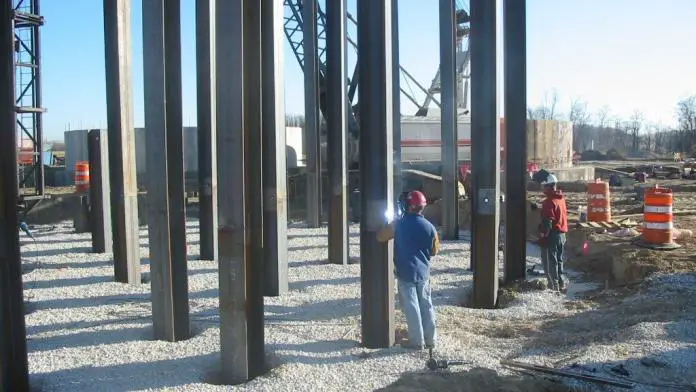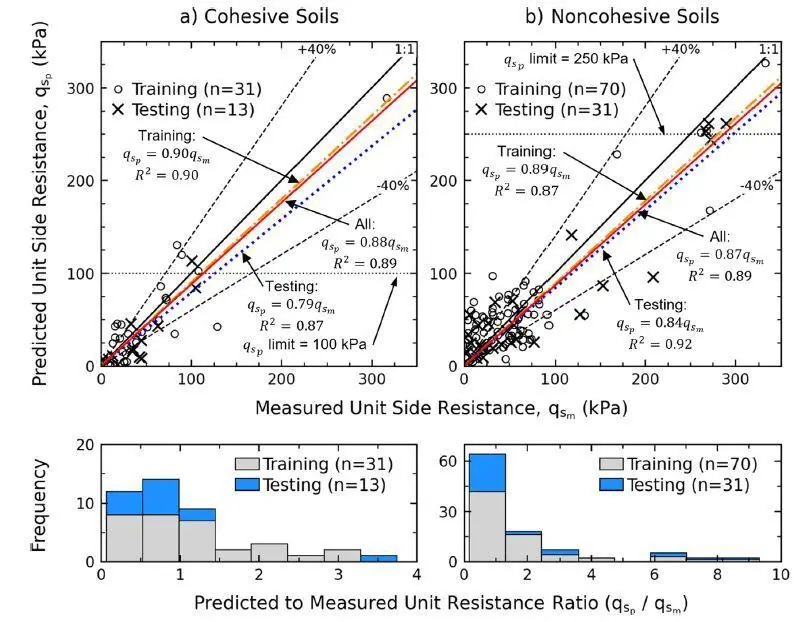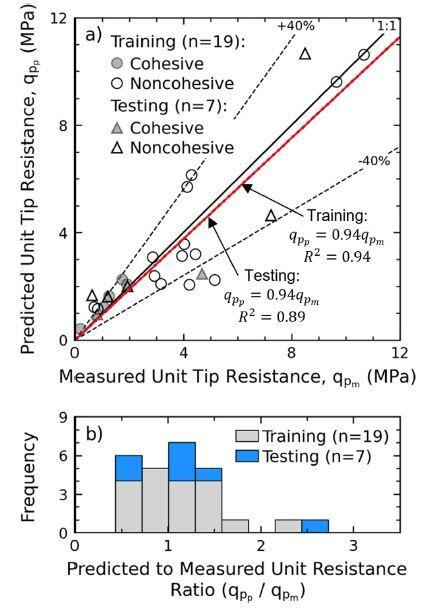# Application of Genetic Algorithm to the Design of Pile FoundationsMachine learning and deep learning techniques have been widely applied in the design of civil engineering structures in recent years. This is mainly due to the development of advanced computational capacity of computers in handling big data. Researchers from Ryerson University, Toronto, Canada have applied the concept of genetic algorithm to the design of pile foundations using Standard Penetration Test (SPT) data. The findings were published in the journal, Soils and Foundations.

Genetic Algorithm (GA) is a method of optimization based on Darwin’s theory of evolution. In nature, chromosomes give an organism its characteristics, and organisms can adapt and evolve to survive and thrive in their environments through reproduction. A GA represents the problem domain as a string or matrix termed a chromosome, then evolves the chromosome through reproduction mechanisms to find a solution.

One of the oldest geotechnical investigation procedures, the standard penetration test (SPT), is still widely utilised around the world. SPT is also used to empirically test the ultimate load-carrying capacity (Qu) for the design of pile foundations, despite its significant shortcomings. There are two types of SPT-based design methods: direct and indirect.

Indirect approaches use SPT blow counts (N-values) to determine the shear strength of soils, such as the frictional angle (ϕ) or undrained shear strength (Cu), and then apply these parameters to the design. Unfortunately, correlating ϕ and Cu with SPT N-values is difficult. Some relationships between ϕ and N-values for sands have been proposed from various parts of the world.

According to the authors (Jesswein and Lui, 2022), it may be preferable to directly correlate SPT N-values to pile shaft (Qs) and tip resistance (Qp) given the uncertainties and additional inaccuracies caused by indirect correlations. However, many direct approaches may produce biased Qu forecasts. This is mainly because they were based on simple linear regressions or trial-and-error procedures with the N-value as the only input, and they likely ignore or misrepresent several factors that influence pile-soil interaction, such as effective stress, excess pore pressure, repacking of soil grains during pile driving, and pile load-transfer response.

This inspired the authors to consider machine learning (ML) approach. ML algorithms are more efficient at regressing huge datasets and capturing nonlinear interactions between numerous variables than standard regression approaches. Furthermore, because the systems evaluate alternative solutions based on a set of criteria, which typically includes an objective or fitness function, they do not require prior knowledge of the problem domain. This eliminates the need to assume a relationship between variables while performing regression.

Due to its “blackbox” nature, the most popular ML method, artificial neural networks (ANN), does not allow the relationships to be stated in a practical formula. As a result, a genetic algorithm (GA) may be a better ML technique since it may provide useful, easy-to-understand functions that represent generic trends.

A total of 72 axial compression tests on driven steel piles were gathered from the literature and the Ministry of Transportation of Ontario (MTO) for the study. The 72 piles selected were divided into two groups based on their measurements.

The load-transfer distribution data were used to extract both the unit shaft and tip resistances in the first group. The GA developed the design approach by correlating these unit resistances with soil data and pile parameters. The new design approach was validated and compared to three existing SPT-based design methods in the second group, where only total capacity measurements were provided. In the study both N60 and (N1)60 are represented by Ncr.

The piles that were researched were chosen based on the following criteria:

• Sufficient information was available on the subsurface ground conditions, particularly the soil classifications and SPT N-values, at the test site;
• Non-organic soils were found along the pile length;
• The pile types were either open-ended pipe (OEP), closed-ended pipe (CEP), and H piles;
• The pile width or diameter and embedment length were available; and
• The load–displacement curves were reported.

Based on a total of 72 full-scale static load tests reported in the study, the new SPT-based formula provides an unbiased prediction with a higher level of accuracy. The proposed design formulas are summarized below:

qs = Ncrσ’ / 2.55L ≤ 100 kPa (for cohesive soils) ——— (1)
(Training R2 = 0.9; Testing R2 = 0.87; All R2 = 0.89)

qs = 2(Ncr + Ncrσ’/L)/L ≤ 250 kPa (for cohesionless soils) ——— (2)
(Training R2 = 0.87; Testing R2 = 0.92; All R2 = 0.89)

qp = Ncrpt‘/7.57 + 82) ≤ 1200 kPa (for all soils)——— (3)
(Training R2 = 0.94; Testing R2 = 0.89; All R2 = 0.92)

Where;
L = Length of pile
σ’ = Effective overburden pressure at the depth considered
Ncr = Corrected SPT Number
qs = shaft resistance
qp = Tip resistance

According to the authors, Equations (1) and (2) can consider the changing ground conditions by using the characteristic values for Ncr and σ’ within a soil layer.Fig. 4. Comparison between Measured and Predicted Unit Side Resistance by Functions from the GA.(Jesswein and Lui, 2022)Fig. 5. Comparison between Measured and Predicted Unit Tip Resistancefrom the GA (Jesswein and Lui, 2022)

Although the proposed formulas provide better predictions than existing design methods, the authors insist that engineering judgement and knowledge of local ground conditions are critical components in correctly designing a pile. The quality of the produced model is dependent on the correctness of the input variables, particularly Ncr, because GA regressions are data driven. Because regressed models may not perform well under extrapolation, the range of the examined variables should be addressed while applying the provided formulas. The proposed approach can be used on a wide range of cohesive and cohesionless soils, but organic soils and soft clays are not recommended.

Article Source:
Jesswein M. and Liu J. (2022): Using a genetic algorithm to develop a pile design method. Soils and Foundations 62(2022)101175. https://doi.org/10.1016/j.sandf.2022.101175

This is an open access article under the CC BY-NC-ND license (http://creativecommons.org/licenses/by-nc-nd/4.0/).